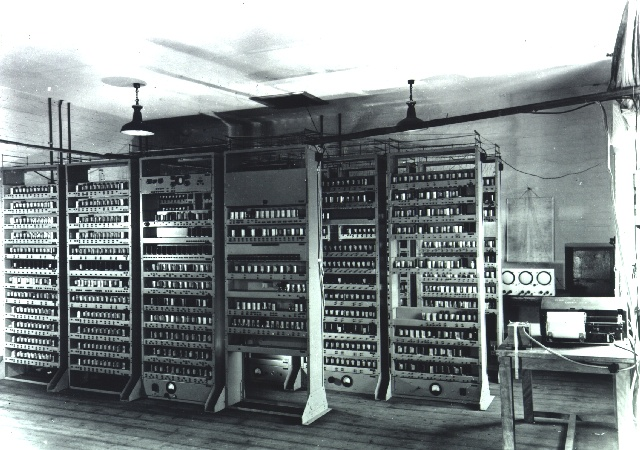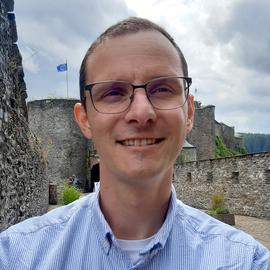# Matching Pursuit Before Computer ScienceI have recently discovered some interesting references about techniques designed around 1938 which could, in my opinion, be considered as (a variant of) the Matching Pursuit algorithm. This is maybe something well known in the literature of the right scientific field, but here is anyway what I recently found.

From what I knew until yesterday, when S. Mallat and Z. Zhang  defined their greedy or iterative algorithm named “Matching Pursuit” (MP) to decompose a signal $$s \in \mathbb{R}^N$$ into a linear combination of atoms picked in a dictionary $$\mathcal{D}=\{g_k\in\mathbb{R}^N: 1\leq k\leq d, g_k^Tg_k = 1\}$$ of $$d$$ elements, they cited a previous method known as “Projection Pursuit” of J. Friedman and W. Tukey  in the field of statistical regression methods.

In short, MP is quite simple. It reads (using matrix notations)

\begin{align} &R^0 = s,\quad A^0 = 0 \tag{initialization}\\\ &R^{n+1}\ =\ R^n\ -\ (g_{*}^T R^n) g_{*},\tag{reconstruction}\\\ &A^{n+1}\ =\ A^n\ +\ (g_{*}^T R^n)g_{*}&\tag{reconstruction} \end{align}

with at each step $$n\geq 0$$ $g_{*} \ = \ {\rm arg max}_{g\in\mathcal{D}}\ |g^T R^n| \tag{sensing}.$ The quantities $$R^n$$ and $$A^n$$ are the residual and the approximation of $$s$$, respectively, at the $$n$$-th step (so also an approximation in $$n$$ terms of $$s$$). A quite direct modification of MP is the Orthogonal Matching Pursuit . In this variant, one still records at each iteration the index (or parameters) of the best atom—the one maximizing its correlation with $$R^n$$, in the “sensing” equation above—but the approximation $$A^n$$ is then computed by a least squares minimization over the set of the $$n$$ previously selected atoms (and not only the last one as in MP).

It is proved in  that MP always converges to … well, something, since one can show that the energy of the residual $$\|R^n\|$$ decreases steadily towards 0 where $$n$$ increases. Under certain assumptions on the dictionary $$\mathcal{D}$$ (such as assuming it to have a small coherence, or cumulative coherence, that roughly measure its closeness to an orthogonal basis) it is also proved that, if $$s$$ is described by a linear combination of few elements of that dictionary (for sparse or compressible signal), i.e., with $$s = \mathcal{D}\alpha_*$$ for a coefficient vector $$\alpha_*\in\mathbb{R}^d$$ with few non-zero (or large) components, then OMP recovers $$\alpha_*$$ in the set of coefficients $$(g_{*}^T R^n)$$ computed at each iteration . For instance, in the trivial case of an orthonormal basis (i.e., with vanishing coherence) (O)MP finds iteratively $$\alpha = \mathcal{D}^{-1} s = \alpha_*$$.

Dozens (or hundreds?) of variations of these initial greedy methods have been introduced since their first formulations in the signal processing community. These variations have improved for instance the initial rate of convergence of MP through the iterations, as well as our ability to solve the sparse approximation problem (i.e., finding the vector $$\alpha_*$$ expressed above). They have also adapted MP to some specific problems like the emerging field of Compressed Sensing. Let’s quote for instance the gradient Pursuit, stagewise OMP, CoSaMP, regularized MP, subspace pursuit, … (see here and here for more information on these algorithms).

Another variation of (O)MP explained by K. Schnass and P. Vandergheynst in , splits the sensing part from the reconstruction part in the initial MP algorithm above, and provides the possibility to select more than only one atom per iteration. In that work, the selection of the best atom $$g_*$$ is performed by a sensing dictionary $$\mathcal{S}$$ while the reconstruction stage, which defines the residuals and the approximations, is still assigned to the first dictionary $$\mathcal{D}$$. This variation is also proved to solve the sparse problem if the two dictionaries satisfy a small cross (cumulative) coherence criterion, which is easier to fulfill than asking for a small (cumulative) coherence of only one dictionary in the initial (O)MP. This reminds the way biorthogonal wavelet bases simplified the selection of wavelets with many moments in wavelet theory.

This last (O)MP variation is precisely the one I discovered it in the separated works of R.V. Southwell  and G. Temple  (the last being more readable in my opinion) written in 1935 and in 1938 (!!), respectively, i.e., before the building of the first (electronic) computers in the 40s.

The context of the method in the initial Southwell’s work was the determination of stresses in structures. Let’s summarize this problem. The structure was modeled by $$N$$ connected springs. If $$\alpha_*\in\mathbb{R}^N$$ represents any motion vector of the springs extremities, then, at the new equilibrium state reached when external forces $$F_{\rm external}\in\mathbb{R}^N$$ are applied to the structure, the internal forces provided by the springs follow, of course, the Hooke law $$F_{\rm internal}=D \alpha_*$$ for a certain symmetric matrix $$D\in\mathbb{R}^{N\times N}$$ containing the spring constants. From Newton’s first law, we thus get $F_{\rm internal} + F_{\rm external} = D \alpha_* + F_{\rm external} = 0.$

In this context, the general question addressed by Southwell was the following. Given a linear system of equations $$D\alpha_* = s$$, with $$s= -F_{\rm external}$$ and $$D$$ positive definite, how can you practically recover $$\alpha_*$$ from $$s$$ and $$D$$? As explained by Temple, a solution to this problem is also “applicable to any problem which is reducible to the solution of a system of non-homogeneous, linear, simultaneous algebraic equations in a finite number of unknown variables”.

Nowadays, the numerical solution seems trivial: take the inverse of $$D$$ and apply it to $$s$$, and if $$D$$ is really big (or even small since I’m lazy) compute $$D^{-1}$$ for instance with Matlab and run >> inv(D)*s (or do something clever with the “/” Matlab operator).

However, the question was raised in the 30s! Even for inverting a ridiculously small 13 × 13 matrix, an analytical solution can be cumbersome to compute. Moreover, it can just be worthless since we are, after all, only interested in finding $$\alpha_*$$, not $$D^{-1}$$. That’s why a few researchers were interested at that time in computing $$A^{-1}s$$, or an approximation to it, without to have this painful inverse computation.

The technique found by R.V. Southwell and generalized later by Temple [4,5], was dubbed of “Successive Relaxation” inside a more general context named “Successive Approximations”. Mathematically, rewriting that work according to the notations of the Matching Pursuit, the Successive Relaxation algorithm reads:

\begin{align} &R^0 = s,\quad \alpha^0 = 0,\tag{initialization}\\\ &R^{n+1}\ =\ R^n\ -\ \beta (R^{n})_{k^*} D_{k^*}\\\ &\alpha^{n+1}\ =\ \alpha^n\ +\ \beta (R^{n})_{k^*} e_{k^*}\tag{reconstruction} \end{align}

where $$\beta\in (0,2)$$, $$D_j$$ is the $$j$$-th column of $$D$$, $$(R^n)_{m}$$ is the $$m$$-th component of $$R^n$$, $$e_j$$ is the vector such that $$(e_j)_k=\delta_{jk}$$ (a canonical basis vector), and with, at each step $$n\geq 0$$, the selection (sensing) $k^{*} = {\rm argmax}_{k} |(R^n)_k|, \tag{sensing}$ i.e., the component of the $$n$$-th residual with the highest amplitude.

In this framework, since $$D$$ is positive definite and thus non-singular, it is proved in  that the vectors $$\alpha^n$$ tend to the true answer $$\alpha_*=D^{-1}s$$. The parameter $$\beta$$ controls the importance of what you removed or add in the residual and in the approximation respectively. You can prove easily that the decreasing of the residual energy is maximum when $$\beta=1$$. In other words, in the concepts introduced in , they designed a Matching Pursuit where they selected for the reconstruction dictionary the orthonormal basis $$D$$ and for the sensing dictionary the identity (the canonical basis) of $$\mathbb{R}^N$$. Amazing, no?

An interesting learning of the algorithm above is the presence of the factor $$\beta$$. In the more general context of (modern) MP with non-orthonormal dictionaries, such a factor could be useful to minimize the “decoherence” effect observed experimentally in the decomposition of a signal when this one is not exactly fitted by elements of the dictionary (as in image processing, where arbitrarily oriented edges have to be described by horizontal and vertical atoms).

G. Temple in  also extended the method to infinite dimensional Hilbert spaces for a different updating step of $$\alpha^n$$. This is nothing else but the foundation of the (continuous) MP studied by authors like R. DeVore and Temlyakov some years ago (on that topic you can read also , a paper I wrote with C. De Vleeschouwer for a geometric description of this continuous formalism).

By googling a bit with the keywords “matching pursuit” and Southwell, I found this presentation of Peter Buhlmann who makes a more general connection between Southwell’s work, Matching Pursuit, and greedy algorithms (around slide 15) in the context of “Iterated Regularization for High-Dimensional Data.” In conclusion, who is this person who explained that we do nothing but always reinventing the wheel? ;-)

If you want to complete this kind of “archeology of Matching Pursuit” please feel free to add some comments below (or to drop me an email). I’ll be happy to read them and improve my general knowledge of the topic.

– Laurent

(text updated on Feb. 15, 2023)

References:

Image credit: EDSAC was one of the first computers to implement the stored program (von Neumann) architecture. Source Wikipedia.